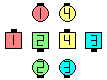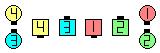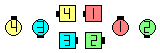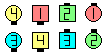Definitions of Square Dance Calls and Concepts

3/4 Tag \$B\$^\$?\$OB>\$NE,@Z\$J(B formation \$B\$+\$i!%(B

Centers \$B\$O(B Trade The Wave, Cast Off 3/4 \$B\$r\$7(B, Counter Rotate 1/4 \$B\$r\$7\$^\$9!%30B&\$N?M\$O(B Cross Cast Back, Touch 1/2 \$B\$r\$7(B, Step & Fold \$B\$r\$7\$^\$9!%(B

\$B1&\$N(B 3/4 Tag \$B\$+\$i\$O(B, \$BJ?9T\$J:8\$N(B Two-Faced Lines \$B\$K\$J\$j(B, \$B:8\$N(B 3/4 Tag \$B\$+\$i\$O(B, \$BJ?9T\$J:8\$N(B Waves \$B\$K\$J\$j\$^\$9!%(B

Cross Counter \$B\$O(B 3 \$B%Q!<%H\$N%3!<%k\$G\$9!%(B

\$BCmLeft \$B\$^\$?\$O(B Mirror \$B\$N;X<(\$,\$J\$1\$l\$P(B, ends \$B\$O(B Touch 1/2 \$B\$G>o\$K1&Cross Counter\$B\$NA0(BCenters Trade The Wave as Outsides Cross Cast Back\$B\$N8e(B Centers Cast Off 3/4 as Outsides Touch 1/2\$B\$N8e(B Centers Counter Rotate 1/4 as Outsides Step & Fold\$B\$N8e(B (\$B=*\$o\$j(B)

Scoot & Cross Counter [C3A]: 1/4 Tag \$B\$+\$i!%(B Scoot Back \$B\$r\$7\$F(B Cross Counter \$B\$r\$7\$^\$9!%(B \$BJ?9T\$J(B Waves \$B\$^\$?\$OJ?9T\$J(B Two-Faced Lines \$B\$G=*\$o\$j\$^\$9!%(B \$B\$3\$l\$O(B 4 \$B%Q!<%H\$N%3!<%k\$G\$9!%(B

Counter [C2] : 3/4 Tag \$B\$^\$?\$OB>\$NE,@Z\$J(B formation \$B\$+\$i!%(B Centers \$B\$O(B Cast Off 3/4 \$B\$r\$7\$F(B Counter Rotate 1/4 \$B\$r\$7\$^\$9!%(B \$B30B&\$N?M\$O(B Cast Back, Touch 1/2, \$B\$=\$7\$F(B Step & Fold \$B\$r\$7\$^\$9!%(B \$B1&\$N(B 3/4 Tag \$B\$+\$i\$O(B, \$BJ?9T\$J:8\$N(B Waves \$B\$K\$J\$j(B, \$B:8\$N(B 3/4 Tag \$B\$+\$i\$O(B, \$BJ?9T\$J:8\$N(B Two-Faced Lines \$B\$K\$J\$j\$^\$9!%(B